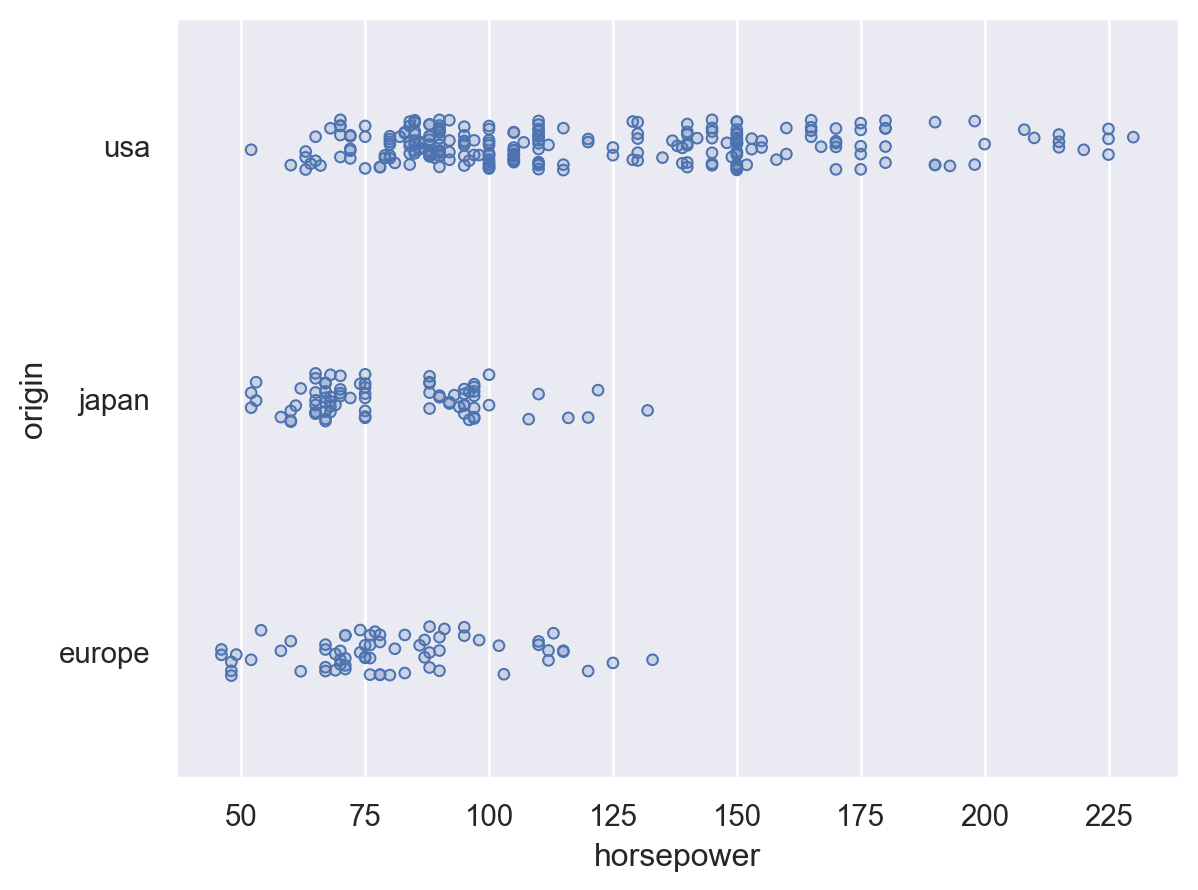# seaborn.objects.Dots#

class seaborn.objects.Dots(artist_kws=<factory>, marker=<rc:scatter.marker>, pointsize=<4>, stroke=<0.75>, color=<'C0'>, alpha=<1>, fill=<True>, fillcolor=<depend:color>, fillalpha=<0.2>)#

A dot mark defined by strokes to better handle overplotting.

This mark defines the following properties:

`Dot`

A mark suitable for dot plots or less-dense scatterplots.

Examples

This mark draws relatively small, partially-transparent dots:

```p1 = so.Plot(mpg, "horsepower", "mpg")
```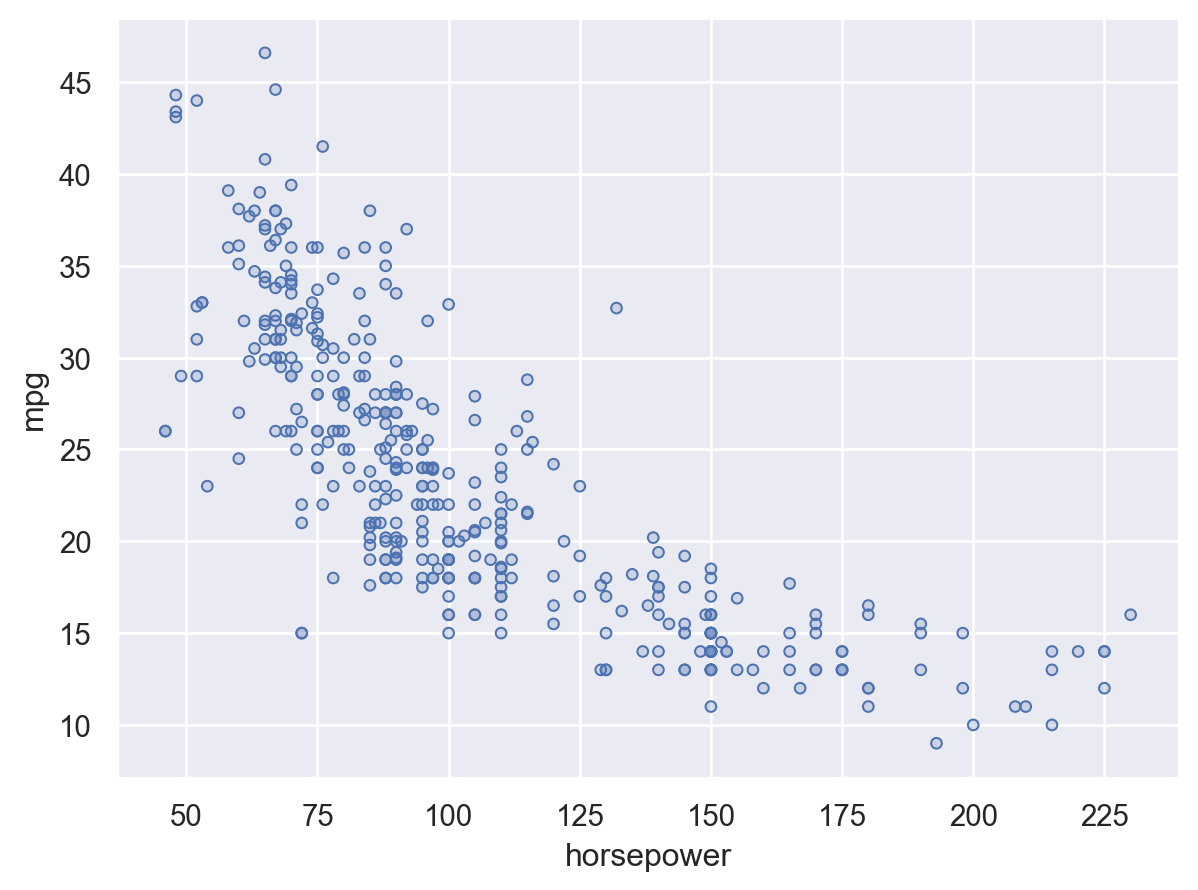Fixing or mapping the `color` property changes both the stroke (edge) and fill:

```p1.add(so.Dots(), color="origin")
```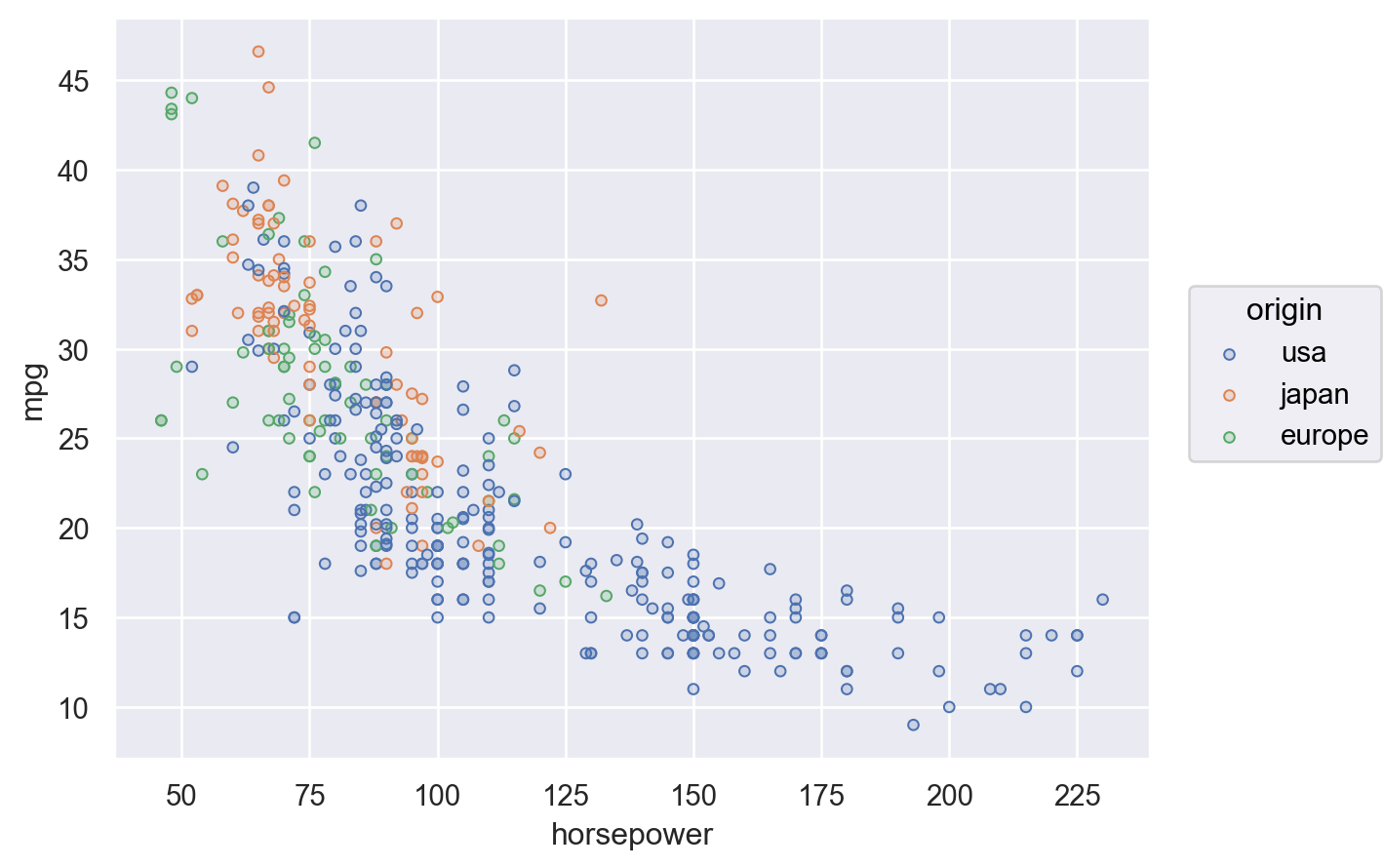These properties can be independently parametrized (although the resulting plot may not always be clear):

```(
.scale(fillcolor="binary")
)
```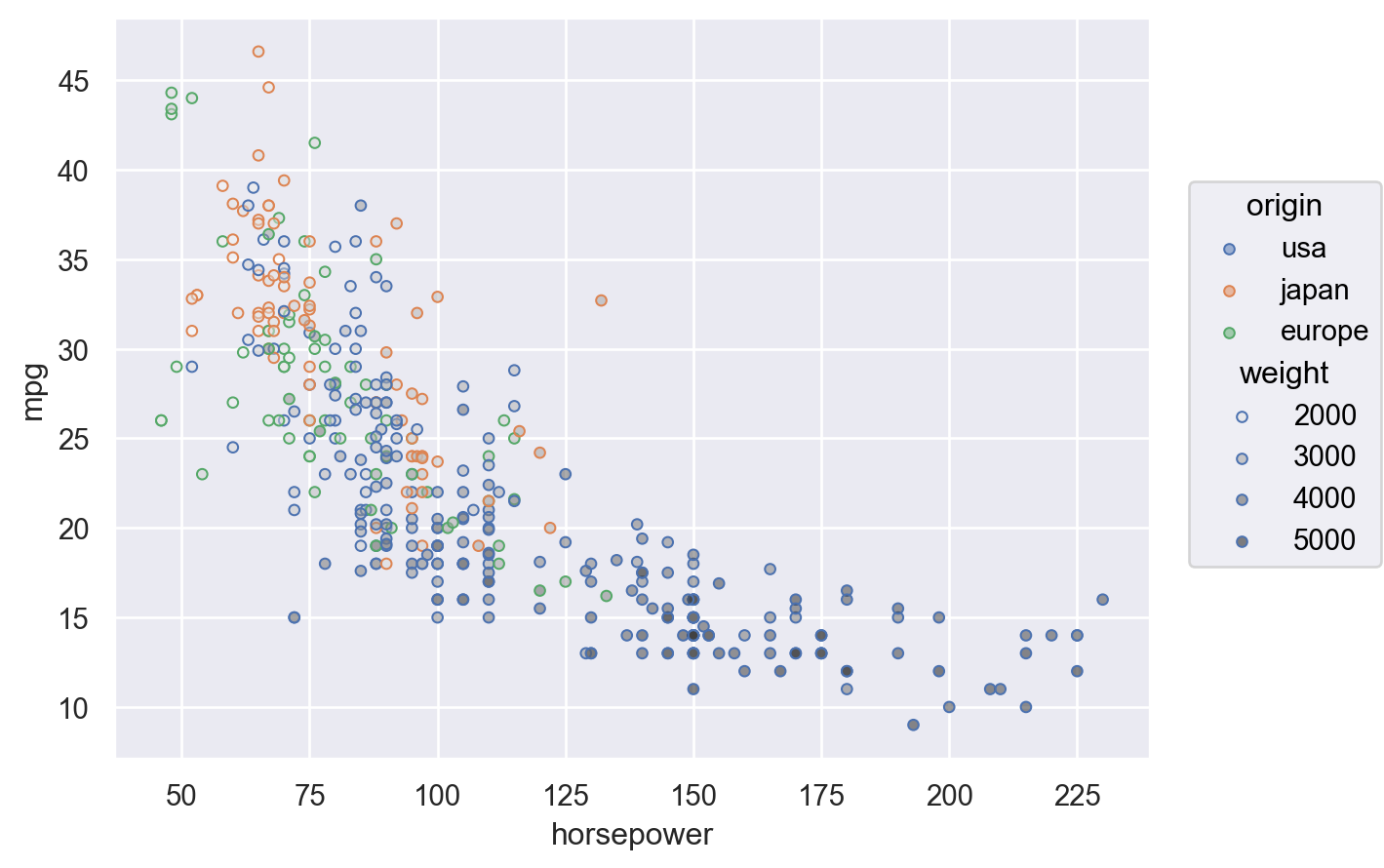Filled and unfilled markers will happily mix:

```p1.add(so.Dots(stroke=1), marker="origin").scale(marker=["o", "x", (6, 2, 1)])
```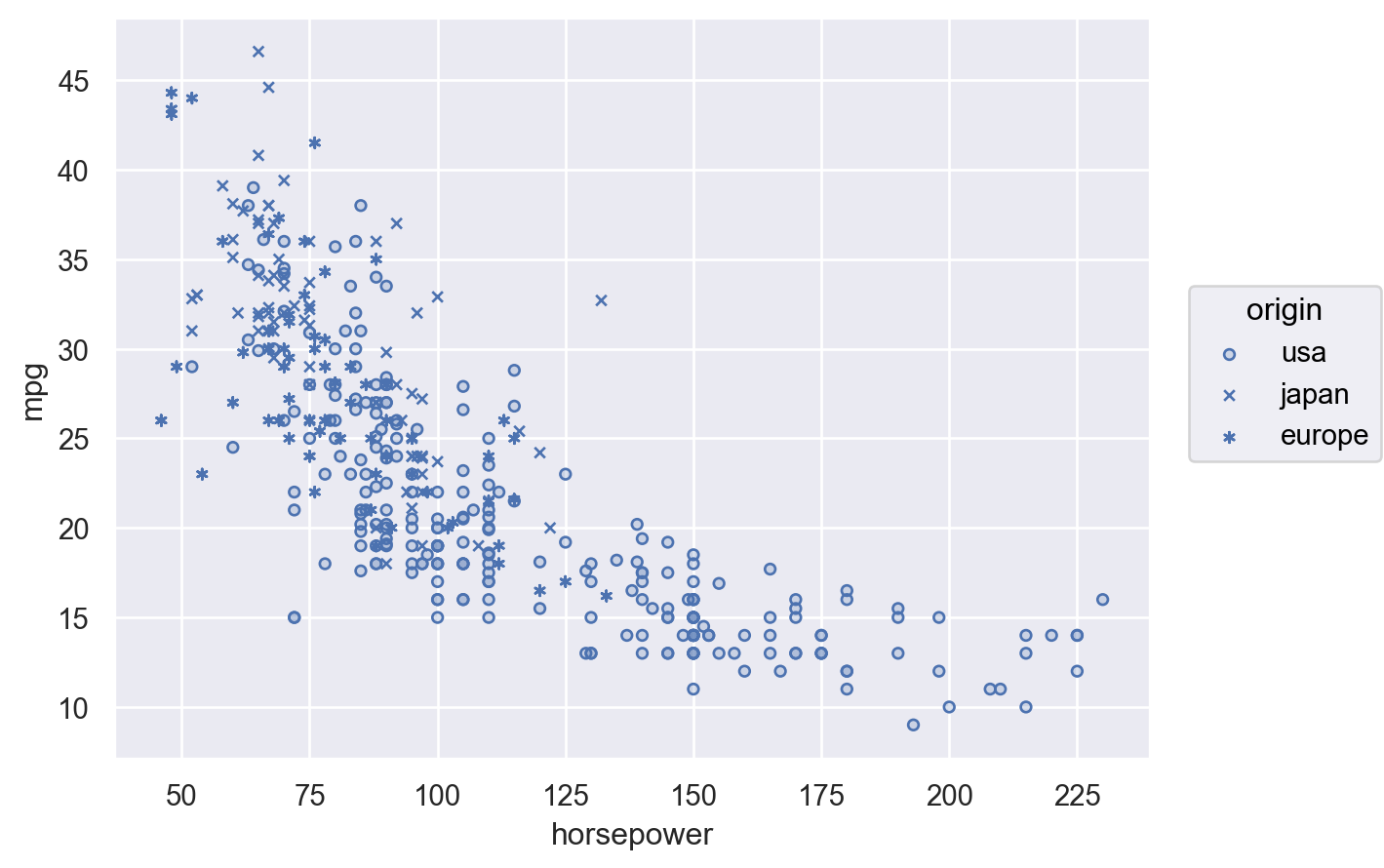The partial opacity also helps to see local density when using jitter:

```(
so.Plot(mpg, "horsepower", "origin")